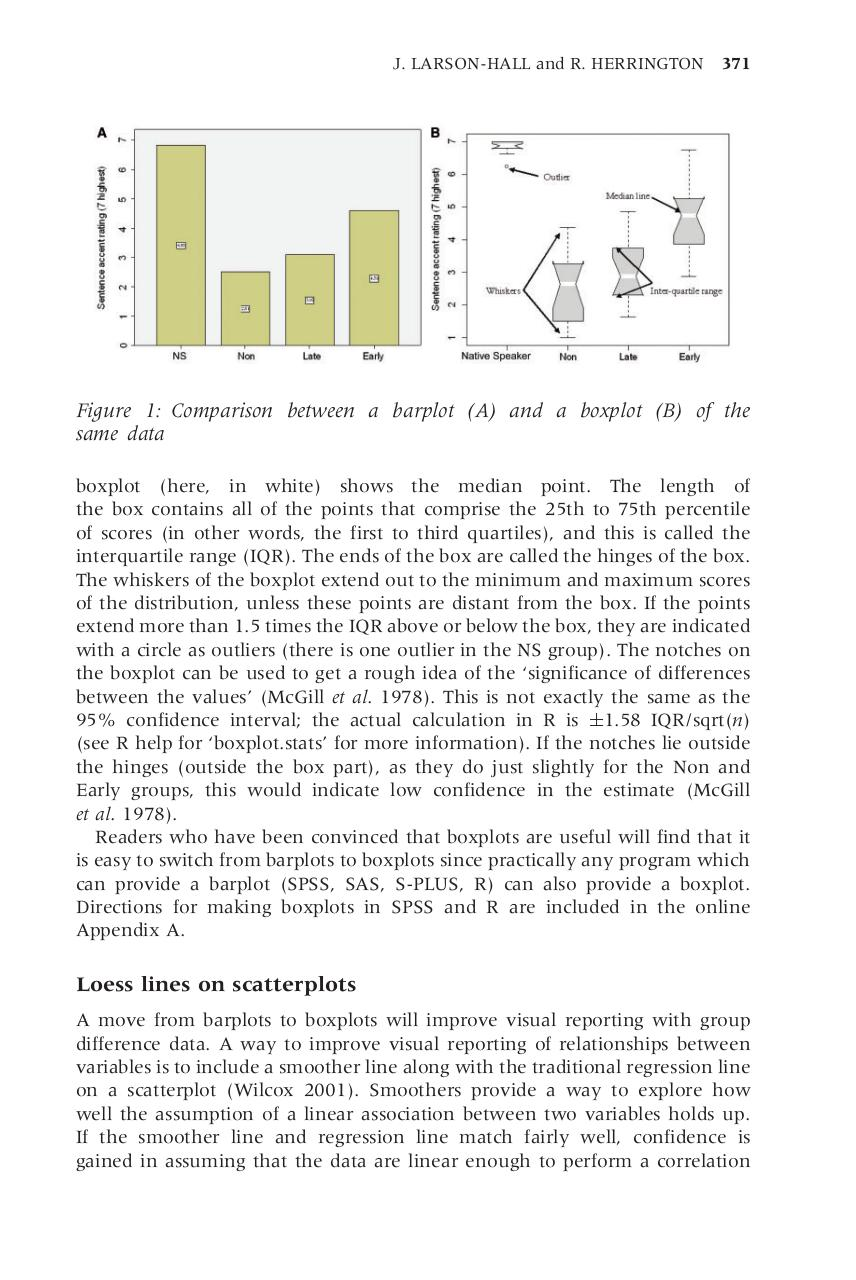10.pdfPage 1 2 3 45624

Text preview

J. LARSON-HALL and R. HERRINGTON

371

Figure 1: Comparison between a barplot (A) and a boxplot (B) of the
same data
boxplot (here, in white) shows the median point. The length of
the box contains all of the points that comprise the 25th to 75th percentile
of scores (in other words, the first to third quartiles), and this is called the
interquartile range (IQR). The ends of the box are called the hinges of the box.
The whiskers of the boxplot extend out to the minimum and maximum scores
of the distribution, unless these points are distant from the box. If the points
extend more than 1.5 times the IQR above or below the box, they are indicated
with a circle as outliers (there is one outlier in the NS group). The notches on
the boxplot can be used to get a rough idea of the ‘significance of differences
between the values’ (McGill et al. 1978). This is not exactly the same as the
95% confidence interval; the actual calculation in R is !1.58 IQR/sqrt(n)
(see R help for ‘boxplot.stats’ for more information). If the notches lie outside
the hinges (outside the box part), as they do just slightly for the Non and
Early groups, this would indicate low confidence in the estimate (McGill
et al. 1978).
Readers who have been convinced that boxplots are useful will find that it
is easy to switch from barplots to boxplots since practically any program which
can provide a barplot (SPSS, SAS, S-PLUS, R) can also provide a boxplot.
Directions for making boxplots in SPSS and R are included in the online
Appendix A.

Loess lines on scatterplots
A move from barplots to boxplots will improve visual reporting with group
difference data. A way to improve visual reporting of relationships between
variables is to include a smoother line along with the traditional regression line
on a scatterplot (Wilcox 2001). Smoothers provide a way to explore how
well the assumption of a linear association between two variables holds up.
If the smoother line and regression line match fairly well, confidence is
gained in assuming that the data are linear enough to perform a correlation